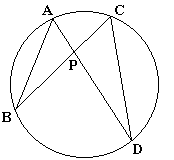# Intersecting Chords Theorem

The Intersecting Chords Theorem asserts the following very useful fact:Given a point P in the interior of a circle, pass two lines through P that intersect the circle in points A and D and, respectively, B and C. Then AP·DP = BP·CP.

The proof follows easily from the similarity of triangles ABP and CDP that is a consequence of the equality of their angles:

 ∠BAD = ∠BCD, as inscribed angles subtended by the same chord BD, ∠ABC = ∠ADC, as inscribed angles subtended by the same chord AC, ∠APB = ∠CPD, as a pair of vertical angles.

(Vertical angles are formed by the same intersecting lines, but the opposite rays - the halves of the lines - cut off by their point of intersection.)

From the similarity of triangles ABP and CDP, we obtain the proportion:

 (*) AP/CP = BP/DP = AB/CD.

The first identity (AP/CP = BP/DP) leads directly to the Intersecting Chords Theorem: AP·DP = BP·CP.

Since the proof only uses the equality of the first two ratios, a keen observer might inquire whether the third ratio (AB/CD) is of any use. The following problem (due to N. Vasil'ev) appeared in the Russian Kvant (M26, March-April, 1991, 30) and was further popularized by R. Honsberger in his Mathematical Chestnuts from Around the World.

Boat 1 and boat 2, which travel at constant speeds, not necessarily the same, depart at the same time from docks A and C, respectively, on the banks of a circular lake. If they go straight to docks D and B, respectively, they collide. Prove that if boat 1 goes instead straight to dock B and boat 2 goes straight to dock D, they arrive at their destinations simultaneously.

The proof follows (*) by involving the third ratio!

First, let v1 and v2 be the speeds of the boats. The fact that they collide if the first is headed from A to D and the second from C to B, means that they arrive at point P simultaneously, i.e.,

AP/v1 = CP/v2.

The latter immediately translates into

AP/CP = v1/v2.

Taking (*) into account, this implies

AB/CD = v1/v2,

or

AB/v1 = CD/v2,

which exactly means that, if boat 1 heads to B and boat 2 heads to D, they arrive there simultaneously.

## References

1. R. Honsberger, Mathematical Chestnuts from Around the World, MAA, 2001, p. 115### Power of a Point wrt a Circle